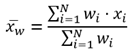# 范围内汇总

##### 注：范围内汇总工具可计算输入图层位于边界图层内或与之重叠区域的统计数据。 您执行范围内汇总的区域可以是面图层、六边形图格或方形图格。

## 工作流示意图## 示例

• 为高效完成例行维护项目，城市将使用范围内汇总计算各维护评估地区内的街灯数量并得出自行车车道的总英里数。 然后即可据其估算出各地区完成该项工作所需的材料和人员情况。

• 一家有线电视提供商即将启动一项试点工程，该工程可使收入较低的社区大学的学生以较低的成本访问互联网。 范围内汇总可用于确定各学区内低收入家庭的数量，以便有线电视提供商可以选择合适的地区来实施其试点工程。

• 开发公司希望为某县城区中心打造新型综合性开发项目。 在各城市中，均可使用范围内汇总计算方便到达商店、饭店或轻轨的潜在开发位置的方形区域。 这样可以简化地点选择流程。

• 英里
• 英尺
• 千米

• 平方英里
• 平方千米
• 平方米
• 公顷
• 英亩

## 范围内汇总工作原理

### 等式### 点

``[280, 408, 356, 361, 450, 713] = 6``

``280 + 408 + 356 + 361 + 450 + 713 = 2,568``

``[280, 408, 356, 361, 450, 713] = 280``

``[280, 408, 356, 361, 450, 713] = 713``

``713 - 280 = 433``

``2568/6 = 428````= 22737.2````= 150.7886``

``= 6``

= 中学

### 线

``2/3 = .3333``

``3/6 = .5``

``[1000, 600] = 2``

``1 x (3/6) + 1 x (2/3) = 1.667``

``1000 + 600 = 1600``
``1000 x (3/6) + 600 x (2/3) = 900``

``[1000, 600] = 600``

``[1000 x (3/6), 600 x (2/3)]``
``[500, 400] = 400``

``[1000, 600] = 1000``

``[1000 x (3/6), 600 x (2/3)]``
``[500, 400] = 500``

``1600 - 600 = 1000``
``500 - 400 = 100``

``(1000 + 600)/2 = 800``
``(1000 x (3/6) + 600 x (2/3))/(3/6 + 2/3)``
``(500 + 400)/(4/6) = 1350````= 80000````= 1268571.4286````= 282.8427````= 1126.3088``

### 面

``4/(2+4) = 3/4``

``4/(2+4) = 2/3``

``1/(1+1.5) = 2/5``

``6/(2+6) = 3/4``

``2/(2+2) = 1/2``

``[3200, 4700, 1000, 4500, 3600] = 5``

``(3/4)+(2/3)+ (2/5)+(3/4)+ (1/2) = 3.06667``

``3200 + 4700 + 1000 + 4500 + 3600 = 17000``
``(3/4) x 3200 +(2/3) x 4700 + (2/5) x 1000 +(3/4) x 4500 + (1/2) x 3600 = 11108.33``

``[3200, 4700, 1000, 4500, 3600] = 1000``

``[(3/4) x 3200, (2/3) x 4700, (2/5) x 1000, (3/4) x 4500, (1/2) x 3600]``
``[2400, 3133.33, 400, 3375, 1800] = 400``

``3200, 4700, 1000, 4500, 3600] = 4700``

``[2400, 3133.33, 400, 3375, 1800] = 3375``

``4700 - 1000 = 3700``
``3,375 - 400 = 2,975``

``(17000)/5 = 3400``
``(11108.33)/[3.06667] = 3622.83````= 2185000````= 1727137.5112````= 1478.175````= 1314.2060``

## ArcGIS API for Python 示例

``````# Import the required ArcGIS API for Python modules
import arcgis
from arcgis.gis import GIS
from arcgis.geoanalytics import summarize_data

# Connect to your ArcGIS Enterprise portal and confirm that GeoAnalytics is supported
portal = GIS("https://myportal.domain.com/portal", "gis_publisher", "my_password", verify_cert=False)
if not portal.geoanalytics.is_supported():
print("Quitting, GeoAnalytics is not supported")
exit(1)

# Find the big data file share dataset you'll use for analysis
search_result = portal.content.search("", "Big Data File Share")

# Look through the search results for a big data file share with the matching name
bdfs_search = next(x for x in search_result if x.title == "bigDataFileShares_CityData")

# Look through the big data file share for BikeLanes
bike_lanes = next(x for x in bdfs_search.layers if x.properties.name == "BikeLanes")

# Look through the big data file share for districts
districts = next(x for x in bdfs_search.layers if x.properties.name == "districts")

weighted_summary_fields = [{"statisticType" : "Average","onStatisticField" : "Slope"}]

# Run the Summarize Within tool
summarize_within_result = summarize_data.summarize_within(summary_polygons = districts,
summarized_layer = bike_lanes,
weighted_summary_fields = weighted_summary_fields,
output_name = "summary_of_bike_lanes")

# Visualize the tool results if you are running Python in a Jupyter Notebook
processed_map = portal.map('Your City, State', 10)
processed_map``````

## 类似工具

### ArcGIS Desktop 分析工具

GeoAnalytics ToolsArcGIS Pro 中可用。